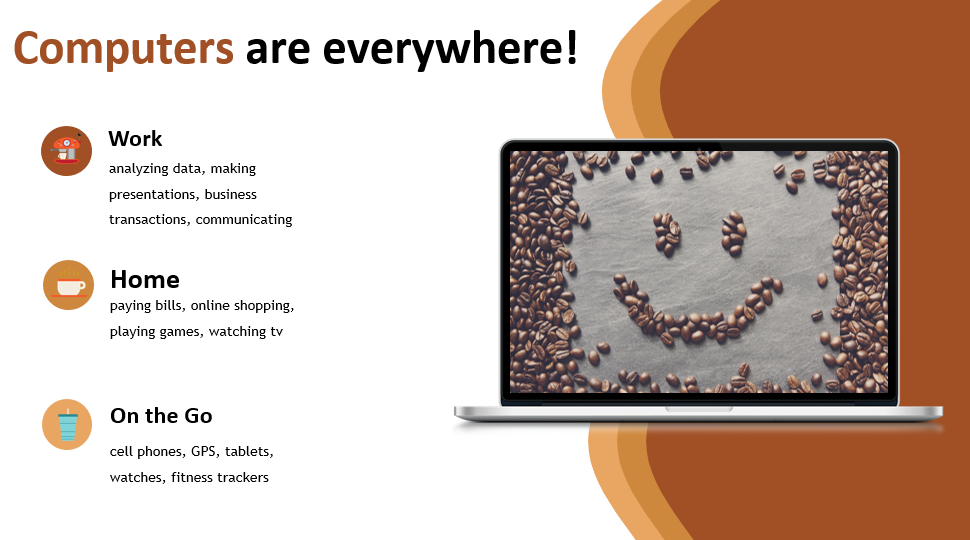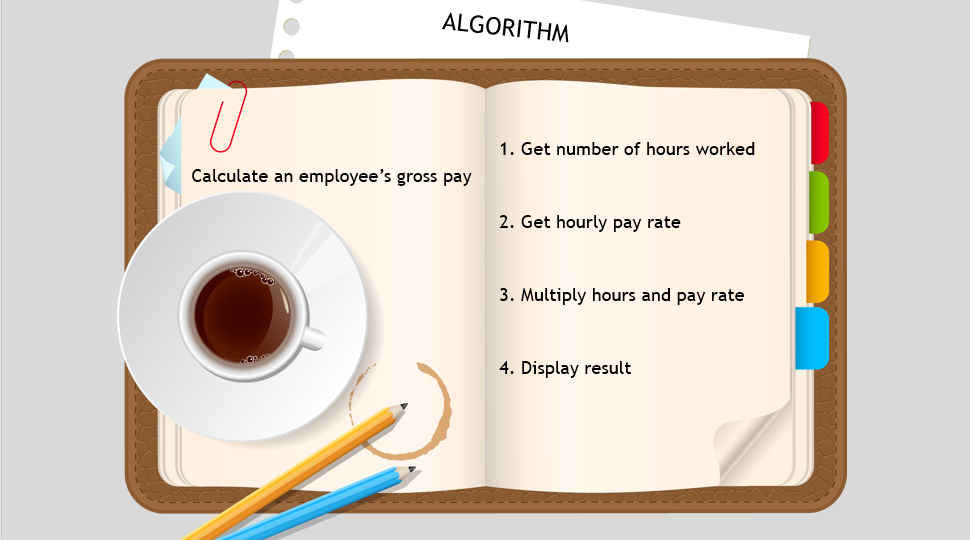Transcripted Summary

In this chapter, we'll cover some fundamentals of computer programming and algorithms.

Computers are a part of our everyday lives. They're everywhere.We use them at work. We use them at home. We use them while we're out on the go. So we're all very familiar with computers.

But from a programmer's standpoint, a computer is simply a machine that is capable of processing data.

Contrary to popular belief, computers are not smart. They're not capable of thinking for themselves, and therefore, they need a program to provide precise instructions on how to do everything.

A program, also known as software, is a set of instructions that a computer follows to perform a task.

Programs were originally written in machine language, a series of 0s and 1s, because this is the only language computers understand. People later created more human-friendly programming languages to write in, such as Java. These languages are translated behind the scenes into machine language, and then interpreted by the computer's hardware. In addition to Java, there are many other programming languages as well.

Given a problem, you can solve that problem using an algorithm, which is a high-level step-by-step guide to complete a task.

You can implement an algorithm using a programming language.

Let's look at a problem, and then come up with an algorithm to solve it. Let's say we need to calculate an employee's gross pay. To solve this, we will write down the step-by-step instructions on how we would go about doing this.1. The first step is we'd get the number of hours worked.
2. Then, we get the hourly pay rate.
3. Next, we multiply the hours and the pay rate.
4. Finally, we would display the result.

We can take this algorithm and then use a programming language to implement it. We'll do that in the next chapter.